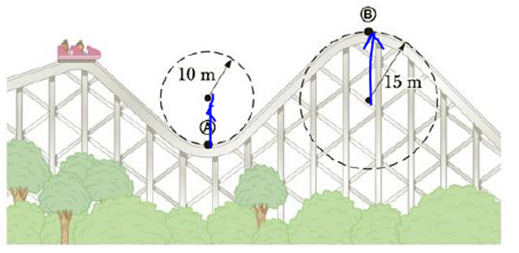Chapter 7, Problem 32P

Chapter
Section
Textbook Problem

A roller-coaster vehicle has a mass of 500 kg when fully loaded with passengers (Fig. P7.32). (a) If the vehicle has a. speed of 200 m/s at point Ⓐ, what is the force of the track on the vehicle at this point? (b) What is the maximum speed the vehicle can have at point Ⓑ for gravity to hold it on the track?Figure P7.32

(a)

To determine
The force of the track on the vehicle at the point A .

Explanation

Given info: The mass of the roller-coaster is 500kg , the radius of the curved path is 10m , and the speed of the roller-coaster at point A is 20.0m/s .

Explanation: Assuming the upward direction of motion as positive and the resultant force on the vehicle is Fy=mac or nmg=mac . The centripetal acceleration of the vehicle ac is v2/r , by using this expression it is easier to calculate the force on the vehicle by the track.

The formula for the force on the vehicle at point is,

n=m(g+vt2r)

• m is mass of the roller-coaster.
• g is acceleration due to gravity.
• vt is tangential speed of the vehicle.
• r is radius of the path at point A

(b)

To determine
The maximum speed the vehicle can have at point B for gravity to hold it on the track.

Still sussing out bartleby?

Check out a sample textbook solution.

See a sample solution

The Solution to Your Study Problems

Bartleby provides explanations to thousands of textbook problems written by our experts, many with advanced degrees!

Get Started

A diet too low in carbohydrate brings about responses that are similar to fasting.

Nutrition: Concepts and Controversies - Standalone book (MindTap Course List)

Explain Millikans oil-drop experiment.

General Chemistry - Standalone book (MindTap Course List)

Why are buffers important for living organisms?

Biology: The Dynamic Science (MindTap Course List)